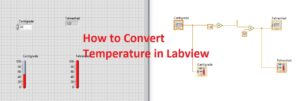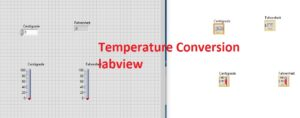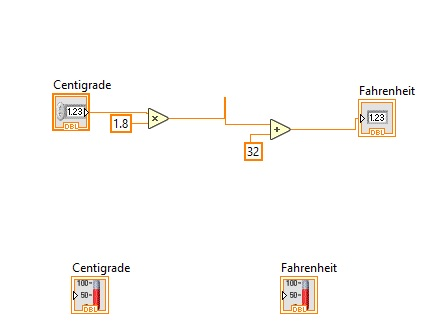Hi reader welcomes to another interesting post. in this post, we will do Temperature Conversion Using LabVIEW. Temperature tells about the degree of coldness and hotness of any substance. These different types of units are used according to material features. but there are 3 main types of units that commonly used, that are Centigrade, Fahrenheit, Kelvin.

In this post, we will make some programs through which we can do the conversion of these temperature units from one to another such as Kelvin to Centigrade, etc. So let’s get started with Temperature Conversion Using LabVIEW.

## Common Temperature Conversion Formulas

• Before making a program to convert these temperatures first of all we see the mathematical expression for these units than make our programs.

Fahrenheit to Celsius Conversion

C = 5/9 ( F – 32 )

Celsius to Fahrenheit Conversion

F = 9/5 °C + 32

Celsius to Kelvin Conversion

K = C + 273

• First of all, we do the centigrade to Fahrenheit conversion.
• For this move to the front panel select the numeric control and numeric indicator.
• Assign the name to numeric control centigrade and numeric indicator to Fahrenheit.
• After that select 2 thermometers and assign them the names centigrade and Fahrenheit.• Now go to block diagram and press right and select the multiply option and link it to centigrade and other terminals write 1.8
• After that select the add option and link it to the multiply and at other terminal write 32.